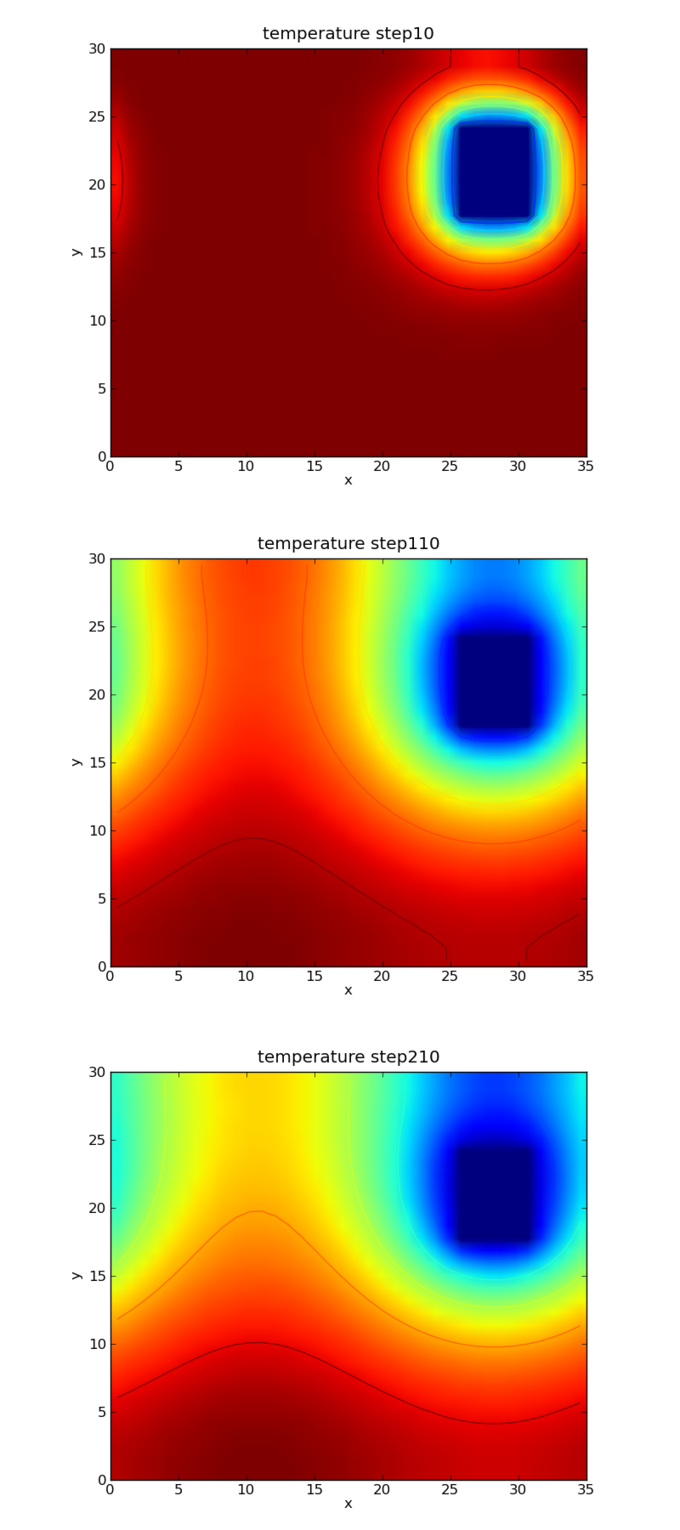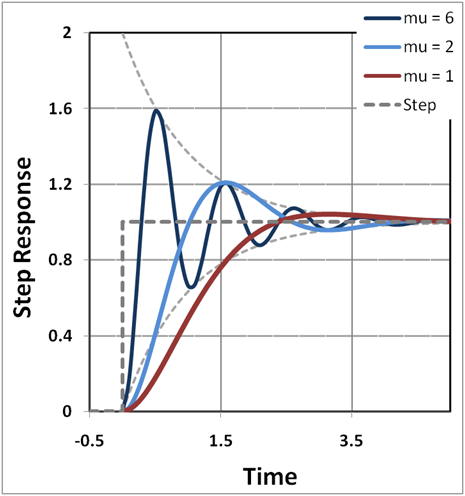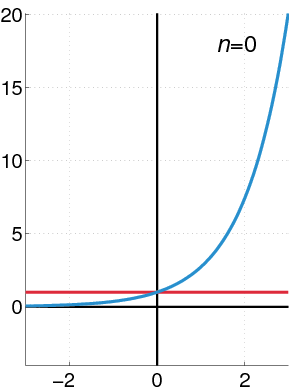## Second-Order Linear Equations

A second-order linear differential equation has the form $\frac{d^2 y}{dt^2} + A_1(t)\frac{dy}{dt} + A_2(t)y = f(t)$, where $A_1(t)$, $A_2(t)$, and $f(t)$ are continuous functions.

### Learning Objectives

Distinguish homogeneous and nonhomogeneous second-order linear differential equations

### Key Takeaways

#### Key Points

• Linear differential equations are of the form $L [y(t)] = f(t)$, where $L_n(y) \equiv \frac{d^n y}{dt^n} + A_1(t)\frac{d^{n-1}y}{dt^{n-1}} + \cdots + A_{n-1}(t)\frac{dy}{dt} + A_n(t)y$.
• When $f(t)=0$, the equations are called homogeneous linear differential equations. (Otherwise, the equations are called nonhomogeneous equations).
• Linear differential equations are differential equations that have solutions which can be added together to form other solutions.

#### Key Terms

• linear: having the form of a line; straight
• differential equation: an equation involving the derivatives of a function

Linear differential equations are of the form $Ly = f$, where the differential operator $L$ is a linear operator, $y$ is the unknown function (such as a function of time $y(t)$), and the right hand side $f$ is a given function of the same nature as $y$ (called the source term). For a function dependent on time, we may write the equation more expressly as $L y(t) = f(t)$ and, even more precisely, by bracketing $L [y(t)] = f(t)$.

The linear operator $L$ may be considered to be of the form:

$\displaystyle{L_n(y) \equiv \frac{d^n y}{dt^n} + A_1(t)\frac{d^{n-1}y}{dt^{n-1}} + \cdots + A_{n-1}(t)\frac{dy}{dt} + A_n(t)y}$

The linearity condition on $L$ rules out operations such as taking the square of the derivative of $y$, but permits, for example, taking the second derivative of $y$. It is convenient to rewrite this equation in an operator form:

$\displaystyle{L_n(y) \equiv \left[\,D^n + A_{1}(t)D^{n-1} + \cdots + A_{n-1}(t) D + A_n(t)\right] y}$

where $D$ is the differential operator $\frac{d}{dt}$ (i.e. $Dy = y'$, $D^2y = y''$, $\cdots$) that is involved.

### Second-Order Linear Differential Equations

A second-order linear differential equation has the form:

$\displaystyle{\frac{d^2 y}{dt^2} + A_1(t)\frac{dy}{dt} + A_2(t)y = f(t)}$

where $A_1(t)$, $A_2(t)$, and $f(t)$ are continuous functions. When $f(t)=0$, the equations are called homogeneous second-order linear differential equations. (Otherwise, the equations are called nonhomogeneous equations.)Simple Pendulum: A simple pendulum, under the conditions of no damping and small amplitude, is described by a equation of motion which is a second-order linear differential equation.

## Nonhomogeneous Linear Equations

Nonhomogeneous second-order linear equation are of the the form: $\frac{d^2 y}{dt^2} + A_1(t)\frac{dy}{dt} + A_2(t)y = f(t)$, where $f(t)$ is nonzero.

### Learning Objectives

Identify when a second-order linear differential equation can be solved analytically

### Key Takeaways

#### Key Points

• Examples of homogeneous or nonhomogeneous second-order linear differential equation can be found in many different disciplines such as physics, economics, and engineering.
• In simple cases, for example, where the coefficients $A_1(t)$ and $A_2(t)$ are constants, the equation can be analytically solved. In general, the solution of the differential equation can only be obtained numerically.
• Linear differential equations are differential equations that have solutions which can be added together to form other solutions.

#### Key Terms

• linearity: a relationship between several quantities which can be considered proportional and expressed in terms of linear algebra; any mathematical property of a relationship, operation, or function that is analogous to such proportionality, satisfying additivity and homogeneity

In the previous atom, we learned that a second-order linear differential equation has the form:

$\displaystyle{\frac{d^2 y}{dt^2} + A_1(t)\frac{dy}{dt} + A_2(t)y = f(t)}$

where $A_1(t)$, $A_2(t)$, and $f(t)$ are continuous functions. When $f(t)=0$, the equations are called homogeneous second-order linear differential equations. Otherwise, the equations are called nonhomogeneous equations. Examples of homogeneous or nonhomogeneous second-order linear differential equation can be found in many different disciplines such as physics, economics, and engineering.Heat Transfer: Phenomena such as heat transfer can be described using nonhomogeneous second-order linear differential equations.

In simple cases, for example, where the coefficients $A_1(t)$ and $A_2(t)$ are constants, the equation can be analytically solved. (Either the method of undetermined coefficients or the method of variation of parameters can be adopted.) In general, the solution of the differential equation can only be obtained numerically. However, there is a very important property of the linear differential equation, which can be useful in finding solutions.

### Linearity

Linear differential equations are differential equations that have solutions which can be added together to form other solutions. If $y_1(t)$ and $y_2(t)$ are both solutions of the second-order linear differential equation provided above and replicated here:

$\displaystyle{\frac{d^2 y}{dt^2} + A_1(t)\frac{dy}{dt} + A_2(t)y = f(t)}$

then any arbitrary linear combination of $y_1(t)$ and $y_2(t)$ —that is, $y(x) = c_1y_1(t) + c_2 y_2(t)$ for constants $c_1$ and $c_2$—is also a solution of that differential equation. This can be confirmed by substituting $y(x) = c_1y_1(t) + c_2 y_2(t)$ into the equation and using the fact that both $y_1(t)$ and $y_2(t)$ are solutions of the equation.

## Applications of Second-Order Differential Equations

A second-order linear differential equation can be commonly found in physics, economics, and engineering.

### Learning Objectives

Identify problems that require solution of nonhomogeneous and homogeneous second-order linear differential equations

### Key Takeaways

#### Key Points

• An ideal spring with a spring constant $k$ is described by the simple harmonic oscillation, whose equation of motion is given in the form of a homogeneous second-order linear differential equation: $m \frac{\mathrm{d}^2x}{\mathrm{d}t^2} + k x = 0$.
• Adding the damping term in the equation of motion, the equation of motion is given as $\frac{\mathrm{d}^2x}{\mathrm{d}t^2} + 2\zeta\omega_0\frac{\mathrm{d}x}{\mathrm{d}t} + \omega_0^{\,2} x = 0$.
• Adding the external force term to the damped harmonic oscillator, we get an nonhomogeneous second-order linear differential equation:$\frac{\mathrm{d}^2x}{\mathrm{d}t^2} + 2\zeta\omega_0\frac{\mathrm{d}x}{\mathrm{d}t} + \omega_0^2 x = \frac{F(t)}{m}$.

#### Key Terms

• damping: the reduction in the magnitude of oscillations by the dissipation of energy
• harmonic oscillator: a system which, when displaced from its equilibrium position, experiences a restoring force proportional to the displacement according to Hooke’s law, where $k$ is a positive constant

Examples of homogeneous or nonhomogeneous second-order linear differential equation can be found in many different disciplines, such as physics, economics, and engineering. In this atom, we will learn about the harmonic oscillator, which is one of the simplest yet most important mechanical system in physics.

### Harmonic oscillator

In classical mechanics, a harmonic oscillator is a system that, when displaced from its equilibrium position, experiences a restoring force, $F$, proportional to the displacement, $x$: $\vec F = -k \vec x \,$, where $k$ is a positive constant. The system under consideration could be an object attached to a spring, a pendulum, etc. Electronic circuits such as RLC circuits are also described by similar equations.

### Simple harmonic oscillation

If $F$ is the only force acting on the system, the system is called a simple harmonic oscillator, and it undergoes simple harmonic motion: sinusoidal oscillations about the equilibrium point, with a constant amplitude and a constant frequency. The equation of motion is given as:

$\displaystyle{F = m a = m \frac{\mathrm{d}^2x}{\mathrm{d}t^2} = -k x}$

Therefore, we end up with a homogeneous second-order linear differential equation:

$\displaystyle{m \frac{\mathrm{d}^2x}{\mathrm{d}t^2} + k x = 0}$

Note that the function $x(t) = A\cos\left( \omega_0 t+\phi\right)$ satisfies the equation where $\omega_0 = \sqrt{\frac{k}{m}} = \frac{2\pi}{T}$. $\omega_0$ is called angular velocity, and the constants $A$ and $\phi$ are determined from initial conditions of the motion.

### Damped harmonic oscillator

In real oscillators, friction (or damping) slows the motion of the system. In many vibrating systems the frictional force $Ff$ can be modeled as being proportional to the velocity v of the object: $Ff = −cv$, where $c$ is called the viscous damping coefficient. Including this additional term, the equation of motion is given as:

$\displaystyle{\frac{\mathrm{d}^2x}{\mathrm{d}t^2} + 2\zeta\omega_0\frac{\mathrm{d}x}{\mathrm{d}t} + \omega_0^{\,2} x = 0}$

where $\zeta = \frac{c}{2 \sqrt{mk}}$ is called the “damping ratio.”Damped Harmonic Oscillators: A solution of damped harmonic oscillator. Curves in different colors show various responses depending on the damping ratio.

Driven harmonic oscillator: Driven harmonic oscillators are damped oscillators further affected by an externally applied force $F(t)$. Newton’s 2nd law ($F=ma$) takes the form:

$\displaystyle{F(t)-kx-c\frac{\mathrm{d}x}{\mathrm{d}t}=m\frac{\mathrm{d}^2x}{\mathrm{d}t^2}}$

It is usually rewritten into the form:

$\displaystyle{\frac{\mathrm{d}^2x}{\mathrm{d}t^2} + 2\zeta\omega_0\frac{\mathrm{d}x}{\mathrm{d}t} + \omega_0^2 x = \frac{F(t)}{m}}$

which is a nonhomogeneous second-order linear differential equation.

## Series Solutions

The power series method is used to seek a power series solution to certain differential equations.

### Learning Objectives

Identify the steps and describe the application of the power series method

### Key Takeaways

#### Key Points

• The power series method calls for the construction of a power series solution $f=\sum_{k=0}^\infty A_kz^k$ for a linear differential equation $f''+{a_1(z)\over a_2(z)}f'+{a_0(z)\over a_2(z)}f=0$.
• The method assumes a power series with unknown coefficients, then substitutes that solution into the differential equation to find a recurrence relation for the coefficients.
• Hermit differential equation $f''-2zf'+\lambda f=0;\;\lambda=1$ has the following power series solution: $f=A_0 \left(1+{-1\over 2}x^2+{-1 \over 8}x^4+{-7 \over 240}x^6+\cdots\right) + A_1\left(x+{1\over 6}x^3+{1 \over 24}x^5+{1 \over 112}x^7+\cdots\right)$.

#### Key Terms

• recurrence relation: an equation that recursively defines a sequence; each term of the sequence is defined as a function of the preceding terms
• analytic functions: a function that is locally given by a convergent power series

The power series method is used to seek a power series solution to certain differential equations. In general, such a solution assumes a power series with unknown coefficients, then substitutes that solution into the differential equation to find a recurrence relation for the coefficients.Maclaurin Power Series of an Exponential Function: The exponential function (in blue), and the sum of the first $n+1$ terms of its Maclaurin power series (in red). Using power series, a linear differential equation of a general form may be solved.

### Method

Consider the second-order linear differential equation:

$a_2(z)f''(z)+a_1(z)f'(z)+a_0(z)f(z)=0$

Suppose $a_2$ is nonzero for all $z$. Then we can divide throughout to obtain:

$\displaystyle{f''+{a_1(z)\over a_2(z)}f'+{a_0(z)\over a_2(z)}f=0}$

Suppose further that $\frac{a_1}{a_2}$ and $\frac{a_1}{a_2}$ are analytic functions. The power series method calls for the construction of a power series solution:

$\displaystyle{f= \sum_{k=0}^\infty A_kz^k}$

After substituting the power series form, recurrence relations for $A_k$ is obtained, which can be used to reconstruct $f$.

### Example

Let us look at the case know as Hermit differential equation:

$f''-2zf'+\lambda f=0\quad (\lambda=1)$

We can try to construct a series solution:

$\displaystyle{f= \sum_{k=0}^\infty A_kz^k \ f'= \sum_{k=0}^\infty kA_kz^{k-1} \ f''= \sum_{k=0}^\infty k(k-1)A_kz^{k-2}}$

substituting these in the differential equation:

\begin{align} & {} \sum_{k=0}^\infty k(k-1)A_kz^{k-2}-2z \sum_{k=0}^\infty kA_kz^{k-1}+ \sum_{k=0}^\infty A_kz^k=0 \\ & = \sum_{k=0}^\infty k(k-1)A_kz^{k-2}- \sum_{k=0}^\infty 2kA_kz^k+ \sum_{k=0}^\infty A_kz^k \end{align}

making a shift on the first sum:

\begin{align} & = \sum_{k+2=0}^\infty (k+2)((k+2)-1)A_{k+2}z^{(k+2)-2}- \sum_{k=0}^\infty 2kA_kz^k+ \sum_{k=0}^\infty A_kz^k \\ & = \sum_{k=0}^\infty (k+2)(k+1)A_{k+2}z^k- \sum_{k=0}^\infty 2kA_kz^k+ \sum_{k=0}^\infty A_kz^k \\ & = \sum_{k=0}^\infty \left((k+2)(k+1)A_{k+2}+(-2k+1)A_k \right)z^k \end{align}

If this series is a solution, then all these coefficients must be zero, so:

$(k+2)(k+1)A_{k+2}+(-2k+1)A_k=0$

We can rearrange this to get a recurrence relation for $A_{k+2}$:

$\displaystyle{A_{k+2}={\frac{(2k-1)}{(k+2)(k+1)}A_k}}$

Now, we have:

$\displaystyle{A_2 = {\frac{-1}{(2)(1)}}A_0={\frac{-1}{2}}A_0, A_3 = {\frac{1}{(3)(2)}} A_1={\frac{1}{6}}A_1}$

and all coefficients with larger indices can be similarly obtained using the recurrence relation. The series solution is:

$\displaystyle{f=A_0 \left(1+\frac{-1}{2}x^2+\frac{-1}{8}x^4+\frac{-7}{240}x^6+ \cdots \right)\\ \,\quad + A_1 \left(x+\frac{1}{6}x^3+\frac{1}{24}x^5+\frac{1}{112}x^7+ \cdots \right)}$